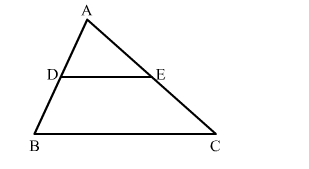# In the given figure, DE∥BC such that AD = x cm, DB = (3x + 4) cm, AE = (x + 3) cm and EC = (3x + 19) cm.

Question:

In the given figure, DE∥BC such that AD = x  cm, DB = (3x + 4) cm, AE = (x + 3) cm and EC = (3x + 19) cm. Find the value of xSolution:

∠ADE = ∠ABC         (Corresponding angles in DE∥BC)
∠AED = ∠ACB         (Corresponding angles in DE∥BC)
By AA similarity criterion, △ADE ∼ △ABC
If two triangles are similar, then the ratio of their corresponding sides are proportional

$\therefore \frac{\mathrm{AD}}{\mathrm{AB}}=\frac{\mathrm{AE}}{\mathrm{AC}}$

$\Rightarrow \frac{\mathrm{AD}}{\mathrm{AD}+\mathrm{DB}}=\frac{\mathrm{AE}}{\mathrm{AE}+\mathrm{EC}}$

$\Rightarrow \frac{x}{x+3 x+4}=\frac{x+3}{x+3+3 x+19}$

$\Rightarrow \frac{x}{4 x+4}=\frac{x+3}{4 x+22}$

$\Rightarrow \frac{x}{2 x+2}=\frac{x+3}{2 x+11}$

$\Rightarrow 2 x^{2}+11 x=2 x^{2}+2 x+6 x+6$

$\Rightarrow 3 x=6$

$\Rightarrow x=2$

Hence, the value of x is 2.